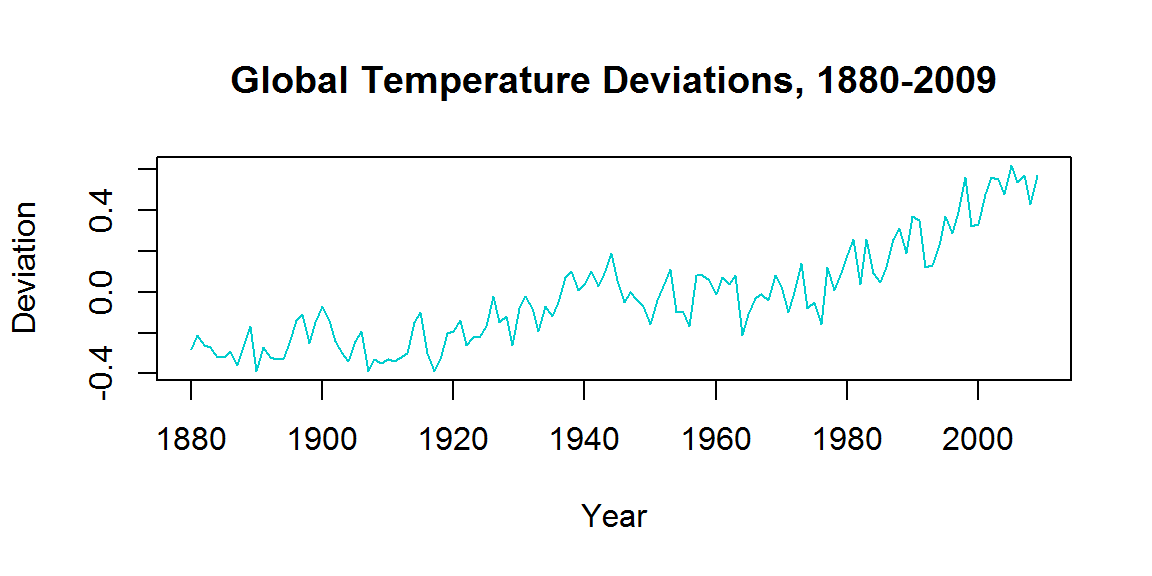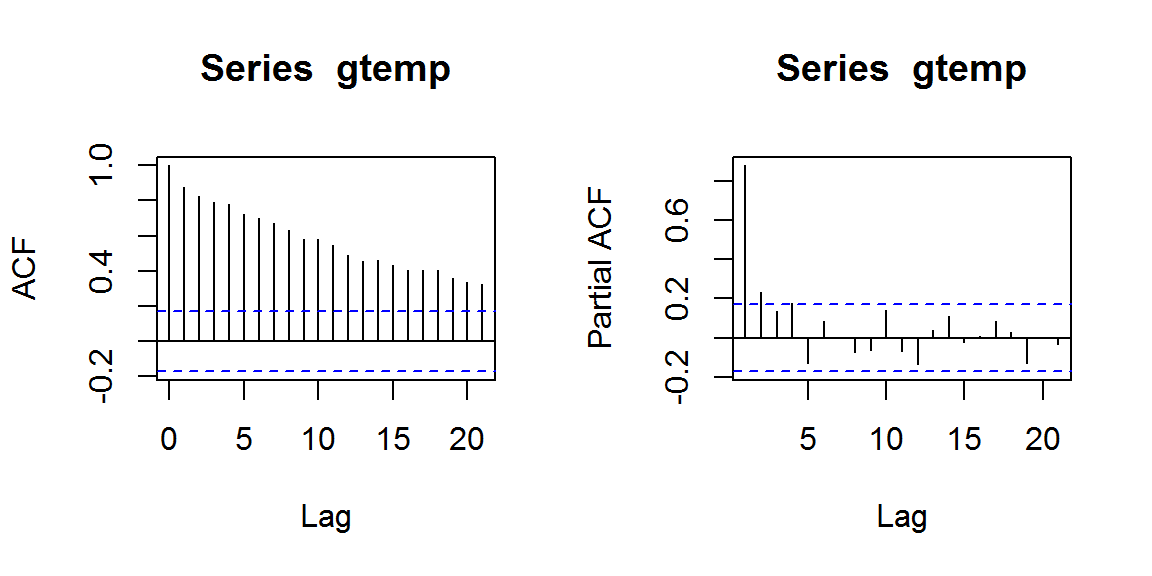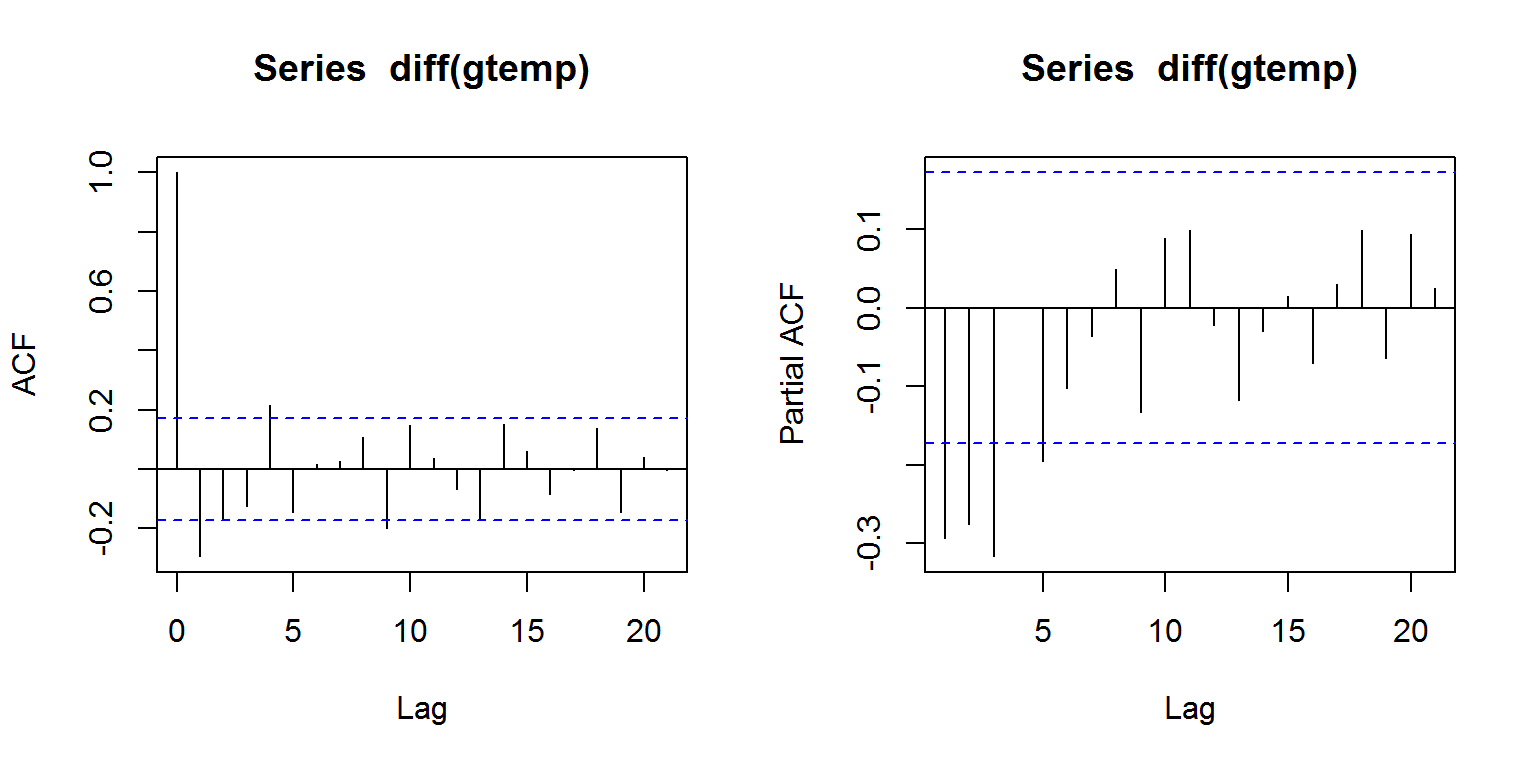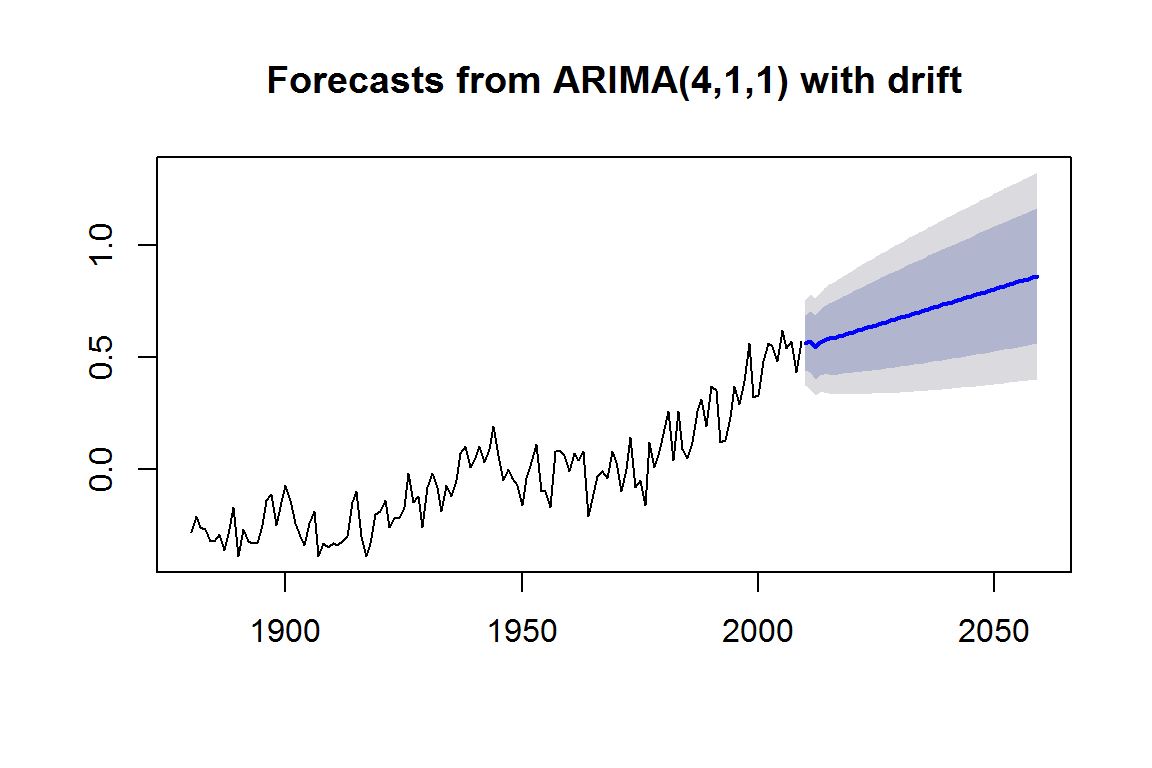### TIME SERIES - CLIMATE CHANGE:

Basically, a reproduction of this post in R-bloggers.The model is not stationary. The criteria for a stationary process are:

1. Constant mean across time.
2. Constant variance across time.
3. Variance between observations only depends on distance (lag).

The variance may be constant, but the mean increases with time.

This can be assessed by observing the number of significant correlations:

par(mfrow=c(1,2))
acf(gtemp)
pacf(gtemp)We can make this series stationary by differencing:

## Loading required package: tseries
adf.test(diff(gtemp), alternative="stationary", k=0)
##
##  Augmented Dickey-Fuller Test
##
## data:  diff(gtemp)
## Dickey-Fuller = -15.158, Lag order = 0, p-value = 0.01
## alternative hypothesis: stationary
par(mfrow = c(1,2))
acf(diff(gtemp))
pacf(diff(gtemp))We can try AR of $$4$$ and MA of $$1$$:

fit1 = Arima(gtemp, order = c(4,1,1), include.drift = T)
future = forecast(fit1, h = 50)
plot(future)